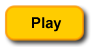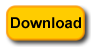# Template Method: Taming Chi SquareEasy Order

Eeeeek! A statistic! Relax, it’s just a Chi Square. The secret to understanding complexity is decomposition and modularity. Decomposition refers to breaking down a whole into its elements. Ironically, the term is fundamental to parallel and concurrent programming where a basic process in programming, like a loop, is further decomposed into more than a single loop, but decomposition also applies to standard OOP programming as well. Any complex process broken down involves the decomposition process. Once broken down, you can attend to the individual modules—modularity.

The Template Method design pattern is a great way to modularize a complex problem, like working through a Chi Square statistic. Chapter 9 in Learning PHP Design Patterns, explains the Template Method in detail and all about “The Hollywood Principle,” but here, I want to look at the Template Method as a “modulaizer”—making complex problems manageable. To get started take a look at Figure 1:Figure 1: 2 x 2 table with row and column totals

Imagine that the data in the table are based on a survey you did at a PHP group, like the Boston group. Suppose that you want to find out the members language preferences based on whether the member owns a Macintosh or a Windows PC. (Sorry Linux.) You’re interested in PHP and jQuery and you record the responses in a two dimensional array. You find that the PC users favor PHP and the Mac users are evenly split between PHP and jQuery. Getting the table results in a 2 x 2 matrix like the one in Figure 1, you’re interested in whether there’s any real significance in the differences or the whole thing is random. You decide to use a Chi Square statistic to find out. Before going further you can run the application and download the files using the buttons below:It Started with a Multi-Dimentional Array

This post came out of a Boston PHP group Percolate session for advanced PHP and OOP programming. Working through Larry Ullman’s PHP Advanced and Object-Oriented Programming, 3rd Ed book, the first chapter covered multi-dimensional arrays. After creating a two-dimensional array, I then put it into a class and started playing with it. You can see it in the following listing:

 ```members= array( array(os => 'Mac', program => 'PHP'), array(os => 'Mac', program => 'jQuery'), array(os => 'PC', program => 'PHP'), array(os => 'PC', program => 'PHP'), array(os => 'PC', program => 'PHP'), array(os => 'PC', program => 'jQuery'), array(os => 'Mac', program => 'jQuery'), array(os => 'Mac', program => 'PHP'), array(os => 'PC', program => 'PHP'), array(os => 'PC', program => 'PHP'), array(os => 'PC', program => 'jQuery'), array(os => 'Mac', program => 'PHP'), array(os => 'PC', program => 'PHP'), array(os => 'Mac', program => 'jQuery'), array(os => 'PC', program => 'PHP'), array(os => 'Mac', program => 'PHP'), array(os => 'PC', program => 'PHP'), array(os => 'PC', program => 'jQuery'), array(os => 'PC', program => 'PHP'), array(os => 'Mac', program => 'jQuery'), array(os => 'Mac', program => 'PHP'), array(os => 'Mac', program => 'jQuery'), array(os => 'PC', program => 'PHP'), array(os => 'PC', program => 'PHP'), array(os => 'PC', program => 'PHP'), array(os => 'PC', program => 'jQuery'), array(os => 'Mac', program => 'jQuery'), array(os => 'Mac', program => 'PHP'), array(os => 'PC', program => 'PHP'), array(os => 'PC', program => 'PHP'), array(os => 'PC', program => 'jQuery'), array(os => 'Mac', program => 'PHP'), array(os => 'PC', program => 'PHP'), array(os => 'Mac', program => 'jQuery'), array(os => 'PC', program => 'PHP'), array(os => 'Mac', program => 'PHP'), array(os => 'PC', program => 'PHP'), array(os => 'PC', program => 'jQuery'), array(os => 'PC', program => 'PHP'), array(os => 'Mac', program => 'jQuery'), array(os => 'Mac', program => 'PHP'), array(os => 'Mac', program => 'jQuery'), array(os => 'PC', program => 'PHP'), array(os => 'PC', program => 'PHP'), array(os => 'PC', program => 'PHP'), array(os => 'PC', program => 'jQuery'), array(os => 'Mac', program => 'jQuery'), array(os => 'Mac', program => 'PHP'), array(os => 'PC', program => 'PHP'), array(os => 'PC', program => 'PHP'), array(os => 'PC', program => 'jQuery'), array(os => 'Mac', program => 'PHP'), array(os => 'PC', program => 'PHP'), array(os => 'Mac', program => 'jQuery'), array(os => 'PC', program => 'PHP'), array(os => 'Mac', program => 'PHP'), array(os => 'PC', program => 'PHP'), array(os => 'PC', program => 'jQuery'), array(os => 'PC', program => 'PHP'), array(os => 'Mac', program => 'jQuery') ); } public function getArray() { return \$this->members; } } ?>```

I wasn’t thinking at all about the Template Method, but when I decided it’d be interesting to do a Chi Square test, I found that the Template Method was both helpful and appropriate. Breaking down the steps in a Chi Square test for a 2 x 2 table is pretty easy:

1. Get the observed cell values (O)
2. Get the column and row totals
3. Determine the expected frequencies (E)
4. Calculate the Chi Square value by adding up (O-E)2 / E
5. Compare the Chi Square value to values in a probability table where df=1 to determine the p value

Those steps must be taken in the order indicated. The exact details of how that is going to be done can vary depending on the data coming in; but the order is set because each step depends on data from the previous step. This is where the template method comes in. It establishes the order in which operations will occur.

Assuming that the client class will read the data from the array to establish the four cell values (O values), the Template Method pattern sets up the sequence for calculating the Chi Square.

 ```public function templateMethod() { \$this->rowColTotals(); \$this->expectedFrequencies(); \$this->calculateX2(); \$this->calcProb(); }```

The methods within the templateMethod() method are abstract. What that means when using the templateMethod() method, the enclosed methods can be implemented in any way necessary. However, they must be executed in the order set up by the templateMethod(). The interface for the Template Method design is an abstract class. The following listing shows the entire class (IChiSquare) housing the templateMethod().

 ```rowColTotals(); \$this->expectedFrequencies(); \$this->calculateX2(); \$this->calcProb(); } } ?>```

To get a broader perspective, Figure 2 shows the Template Method class diagram as implemented for use with Chi Square in this example:Figure 2: Implemented Template Method class diagram.

The Chi Square Implementation

With the template method established in the IChiSquare abstract class, it’s time to implement the ChicSquare class. This is the class that does the actual calculations:

 ```cell1=\$cell1; \$this->cell2=\$cell2; \$this->cell3=\$cell3; \$this->cell4=\$cell4; \$this->templateMethod(); \$this->bundleArray(); return \$this->bundle; }   protected function rowColTotals() { //Row and Column totals \$this->col1total=\$this->cell1 + \$this->cell3; \$this->col2total=\$this->cell2 + \$this->cell4; \$this->row1total=\$this->cell1 + \$this->cell2; \$this->row2total=\$this->cell3 + \$this->cell4; \$this->totalAll=\$this->cell1 + \$this->cell2 + \$this->cell3 + \$this->cell4; }   protected function expectedFrequencies() { //Expected (e) frequencies \$this->e1=(\$this->col1total/\$this->totalAll) * \$this->row1total; \$this->e2=(\$this->col2total/\$this->totalAll) * \$this->row1total; \$this->e3=(\$this->col1total/\$this->totalAll) * \$this->row2total; \$this->e4=(\$this->col2total/\$this->totalAll) * \$this->row2total; }   protected function calculateX2() { //Calculate Chi Square \$x1=pow((\$this->cell1 - \$this->e1),2)/\$this->e1; \$x2=pow((\$this->cell2 - \$this->e2),2)/\$this->e2; \$x3=pow((\$this->cell3 - \$this->e3),2)/\$this->e3; \$x4=pow((\$this->cell4 - \$this->e4),2)/\$this->e4; \$this->chiSquare=\$x1+\$x2+\$x3+\$x4; } protected function calcProb() { //Calculate probability switch(\$this->chiSquare) { case \$this->chiSquare >= 6.64: \$this->p="Significant at .01 level of probability."; break;   case \$this->chiSquare >=3.84: \$this->p="Significant at .05 level of probability."; break;   default: \$this->p="Not significant at .05 or .01 level"; } }   protected function bundleArray() { \$this->bundle=array( "col1t"=>\$this->col1total, "col2t"=>\$this->col2total, "row1t"=>\$this->row1total, "row2t"=>\$this->row2total, "total"=>\$this->totalAll, "x2"=>\$this->chiSquare, "prob"=>\$this->p ); } } ?>```

The great bulk of the ChiSquare class is used up implementing the four key methods from the IChiSquare abstract class. The doX2() method passes arguments from the client and returns the values generated back to the client. The bundleArray() method places all of the calculated value into a one-dimentional associative array so that it can be used by the client to create the table and chi square and probability values.

For me, one of the coolest features of this pattern and the ChiSquare class in particular is how the templateMethod() is called. All of the methods to calculate chi square and the required elements for the table are generated by the implemented methods. So the same pattern could be reused for different tables, requiring only that the methods be expanded to include the table dimensions. As it is, it can handle any 2 X 2 table (df=1). All it needs is the values of the four cells.

The Hard Working Client

Generally, I prefer the client class to do as little as possible other than making requests. In this case, the client class, ChiSqClient is worked harder than a rented mule. It first has to calculate the cell values from the two-dimensional array, and then it passes the cell values to the ChiSquare class and gets the returned values. Finally, it has to build and display a table using the appropriate values. Helper classes could deal with some of that work, but in this case it seemed that a single client class would make it a little easier to see what’s going on.

 ```makeMatrix(\$this->members=\$twoDim->getArray()); \$this->calcX2(); \$this->makeTable(); } private function makeMatrix(array \$twoByTwo) { \$top=count(\$twoByTwo); for (\$row=0; \$row < \$top; \$row++) { if(\$this->members[\$row]["os"]=="PC" && \$this->members[\$row]["program"]=="PHP") { \$this->matrix1++; }   if(\$this->members[\$row]["os"]=="PC" && \$this->members[\$row]["program"]=="jQuery") { \$this->matrix2++; }   if(\$this->members[\$row]["os"]=="Mac" && \$this->members[\$row]["program"]=="PHP") { \$this->matrix3++; } if(\$this->members[\$row]["os"]=="Mac" && \$this->members[\$row]["program"]=="jQuery") { \$this->matrix4++; } } }   private function calcX2() { \$this->x2=new ChiSquare(); \$this->stats=\$this->x2->doX2(\$this->matrix1,\$this->matrix2,\$this->matrix3,\$this->matrix4); }   private function makeTable() { \$c1=\$this->stats["col1t"]; \$c2=\$this->stats["col2t"]; \$r1=\$this->stats["row1t"]; \$r2=\$this->stats["row2t"]; \$total=\$this->stats["total"]; \$chi2= \$this->stats["x2"]; \$p1=\$this->stats["prob"];   \$matrixTable=<< Matrix Table
PHP jQuery
PC \$this->matrix1 \$this->matrix2 \$r1
Mac \$this->matrix3 \$this->matrix4 \$r2
\$c1 \$c2 \$total
Chi Square \$chi2
\$p1;   MATRIX; echo \$matrixTable; } } \$worker=new ChiSqClient(); ?>```

The Template Method design pattern has Swiss Army Knife features in that it is simple yet has many uses. If you have Learning PHP Design Patterns, take a look at Chapter 9 for more details about this handy pattern; especially the materials on the “Hollywood Principle.” In the meantime, play with this implementation and let me know if you have ideas for improvement.

Copyright © 2013 William Sanders. All Rights Reserved.

• No Comments